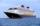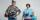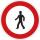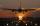# Flying

The airplane from Prague to Bratislava was flying at a speed of 60 km/h less and back by 70 km/h greater than the original speed. What was the original speed if the plane returned to Prague according to the timetable?

Result

v =  840 km/h

#### Solution:Leave us a comment of example and its solution (i.e. if it is still somewhat unclear...):

Showing 0 comments:Be the first to comment!#### To solve this example are needed these knowledge from mathematics:

Looking for help with calculating harmonic mean? Looking for a statistical calculator? Looking for help with calculating roots of a quadratic equation? Do you have a linear equation or system of equations and looking for its solution? Or do you have quadratic equation?

## Next similar examples:

1. The cruise shipThe cruise ship has a speed of 12 km / h at a calm surface. When we sail 45 km along the river and 45 km back, it took us exactly 8 hours. Which (constant) speed of flow of the river?
2. KlaraKlara and Jitka went on a hiking trip at 13 o'clock at speed 5km/h. At 14 o'clock, Tomas ride on the bike at an average speed of 28 km/h. How many hours and at what distance from the beginning of the road Tomáš caught the two girls?
3. Warehouse carsFrom the warehouse started truck at speed 40km/h. After 1hour 30mins started from the same place same direction a car at speed 70 km/h. For how long and at what distance from the warehouse overtake a truck?
4. Holidays - on poolChildren's tickets to the swimming pool stands x € for an adult is € 2 more expensive. There was m children in the swimming pool and adults three times less. How many euros make treasurer for pool entry?
5. Theorem proveWe want to prove the sentense: If the natural number n is divisible by six, then n is divisible by three. From what assumption we started?
6. Expression with powersIf x-1/x=5, find the value of x4+1/x4
7. Square root 2If the square root of 3m2 +22 and -x = 0, and x=7, what is m?
8. Evaluation of expressionsIf a2-3a+1=0, find (i)a2+1/a2 (ii) a3+1/a3
9. AlgebraX+y=5, find xy (find the product of x and y if x+y = 5)
10. PedestrianPedestrian came started at 8h in the morning with speed 4.4 km/h. At half-past eleven cyclist started at 26 km/h same way. How many minutes take cyclist to catch up pedestrian?
11. Wind driftThe plane flies at 860 km/h, passing distance 3000 kilometers with the wind and once again against the wind for 6 h 59 min. What is the wind speed?
12. Fifth of the numberThe fifth of the number is by 24 less than that number. What is the number?
13. Solve 3Solve quadratic equation: (6n+1) (4n-1) = 3n2
14. EquationEquation ? has one root x1 = 8. Determine the coefficient b and the second root x2.
15. FractionFor what x expression ? equals zero?
16. DiscriminantDetermine the discriminant of the equation: ?
17. RootsDetermine the quadratic equation absolute coefficient q, that the equation has a real double root and the root x calculate: ?